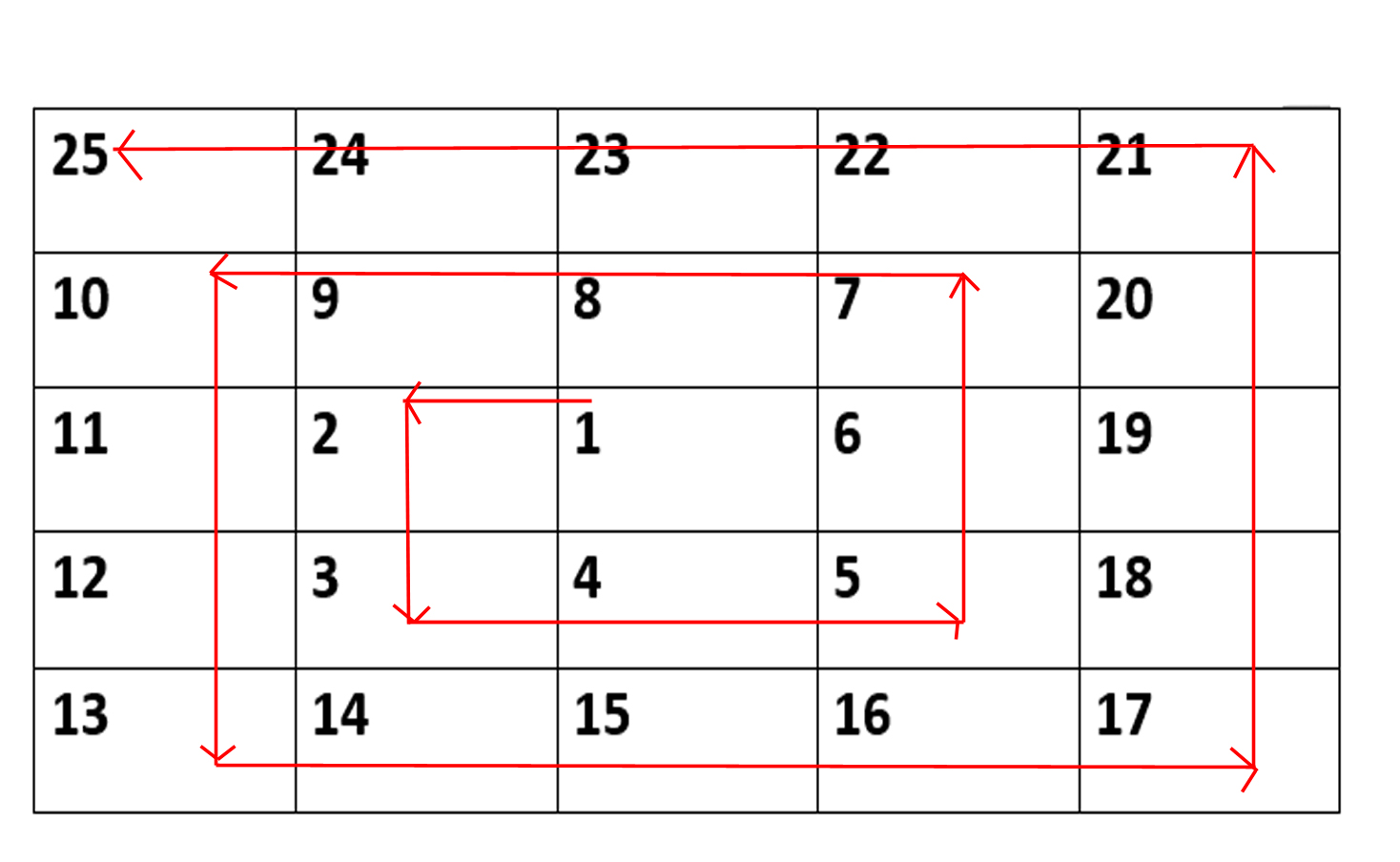# Microsoft Interview experience | Set 107 (On-Campus for Internship)

• Difficulty Level : Hard
• Last Updated : 08 Feb, 2018

Round 1: Coding
Q.1 A 5*5 matrix of spiral order isNow for an N*N spiral matrix filled similar to one given above, find element present at [R,C] position where R=row’s number, C=column’s number.
Constraint: You can’t create matrix itself. Print number without creating matrix explicitly.

Q2. Given a string S and two indexes i & j, modify the string in a pattern such that:
characters from [0 to i] index are now at back of the string.
characters from [j till end of string] are in front of string.
e.g. S = “abcdef” and i=1, j=3.
Output should be: “defcab”
Constraint: Constant Space.

Q3. https://leetcode.com/problems/simplify-path/

Round 2: Group Flyer
Q1. Given an array of positive integers, sort the array in a manner such that when all the elements of new array are concatenated in a string, the number formed is maximum.
e.g. input: [12,9,32]
output: [32,9,12]

Q2. In a native language alphabets are in this order:
a b d c k l g h ng q r
given two string str1 and str2, find which one is larger among the 2. (larger means rankwise more e.g. if “da” and “c” are given then “da” > “c” as d comes first).
write this function:

```   bool compare(char* str1, char* str2);
return 1, if str1 > str2.
return -1, if str2 > str1
return 0, otherwise ```

Interviews: We needed to write our code on a paper.

First F2F round:

Q. https://leetcode.com/problems/letter-combinations-of-a-phone-number/
I did it using recursion and then he asked me to think about iterative approach. One que of DBMS, I told him I am from ECE and haven’t studied DBMS. Then he asked what courses I have done from CSE dept. I told him OOP, DS, Architecture, Network.
Second F2F Round:

Q1. https://www.geeksforgeeks.org/find-longest-path-directed-acyclic-graph/
He gave me this que and asked to solve it in 45 minutes. First he asked about approach. I told him the approach and procedure how I am going to code it.

Then he changed the question I don’t know why and next ques was:
Q2. In a degree ‘N’ tree. Find LCA of 2 given nodes. All elements are unique in tree.
First I gave him a general solution. He didn’t seem convinced because of space complexity and told me to think more. I answered approach in which we can store path from root node to given nodes and match the path. He was convinced then and told me to wait for 5 minutes before 3rd round.

3rd F2F Round:

Q1. Given 2 numbers ‘N’ and ‘M’ where ‘N’ > ‘M’ and last a range of indexs [i,j] between (1,32) which denotes indexes in binary representation of ‘N’, change all the bits in this range to bits of number ‘M’. Also (j-i) is equal to most significant bit of ‘M’
e.g. N=9 :: (binary) 000000…0001001
and M=2 :: (binary) 000000…0000010
and (i=1,j=2) implies 1 to 2 bits of N has to be changed such that they are equal to M’s bits.
expected output: 10 :: (binary) 000000…0001010
where (…) = 19 zeros
First I didn’t get it but then he explained with above example.
Answered it in O(32) in second go.

Q2. Populate next pointer in a binary tree in O(1) space.

Connect nodes at same level using constant extra space

Q3. Something about Deadlock. I asked about the subject and He said OS. I told him I haven’t studied this. He calmly said “Ohh Okay no problem” 😛

Finally someone from their team told me “We are done for the day, You can leave” 😛

Total 11 students were selected.

If you like GeeksforGeeks and would like to contribute, you can also write an article and mail your article to contribute@geeksforgeeks.org. See your article appearing on the GeeksforGeeks main page and help other Geeks.

My Personal Notes arrow_drop_up